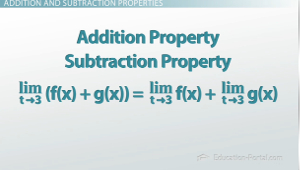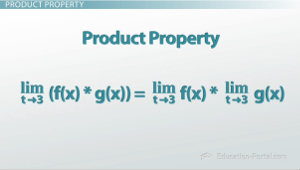# Understanding the Properties of Limits

An error occurred trying to load this video.

Try refreshing the page, or contact customer support.

Coming up next: Squeeze Theorem: Definition and Examples

### You're on a roll. Keep up the good work!

Replay
Your next lesson will play in 10 seconds
• 0:06 Properties of Limits
• 2:32 Product Property
• 3:26 Division Property
• 3:56 Lesson Summary
Save Save

Want to watch this again later?

Timeline
Autoplay
Autoplay
Speed Speed Audio mode

#### Recommended Lessons and Courses for You

Lesson Transcript
Instructor: Jeff Calareso

Jeff teaches high school English, math and other subjects. He has a master's degree in writing and literature.

Graphically we can see limits, but how do we actually calculate them? Three words: Divide and Conquer. In this lesson, explore some of the properties that we can use to find limits.

## Properties of Limits

Let's say that we need to find the limit of f(x) as x goes to some number, like 3. Recall that a limit is what f(x) is going to approach as x goes to 3. This limit can be one or two-sided. If you go from the left side and it's equal to one thing, and if you go from the right side and it's equal to something else, then it's a one-sided limit. If these two values are the same, then it's a two-sided limit.Let's try to find the limit of f(x) = 3x^2 - 1. Well, I can just graph that to try to find the limit as x goes to something like 3. What about something like f(x) = 3(x^2 - 1)(x + 1)^2(3x - 4x^(-1))sin(x)tan(x) … Other than graphing it, or calculating numbers explicitly, how can we find the limit?

Before we actually get into finding the limit, let's take a look at some of the properties that might help us find limits. The first properties of limits are fairly straightforward. These are the addition property and subtraction property. They say that the limit of some sum, like f(x) + g(x), as x goes to some number, like 3, is equal to the limit, as x goes to that number, 3, of f(x) plus the limit, as x goes to 3, of g(x). You've got the first function plus the second function. So this means that we can find the limits separately and just add them together.

Let's consider our function h(x) = x + 3. What is the limit, as x goes to 3, of h(x)? I can use the property of addition to say that the limit of x + 3 as x goes to 3 is equal to the limit of x as x goes to 3, plus the limit of 3 as x goes to 3. In this case, I'd find that the limit is 6.To unlock this lesson you must be a Study.com Member.

### Register to view this lesson

Are you a student or a teacher?

#### See for yourself why 30 million people use Study.com

##### Become a Study.com member and start learning now.
Back
What teachers are saying about Study.com

### Earning College Credit

Did you know… We have over 200 college courses that prepare you to earn credit by exam that is accepted by over 1,500 colleges and universities. You can test out of the first two years of college and save thousands off your degree. Anyone can earn credit-by-exam regardless of age or education level.